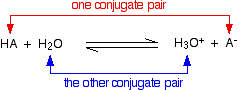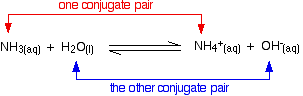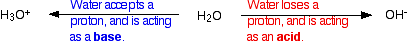Analía Bellizzi – Chemistry Classes

Ronald Reagan Senior High School

# Acid-bases-salt Notes

## Acids and Bases Theory

Introduction:

Water

Water molecule works like a magnet. It has a negative pole and a positive pole. That’s why we say that water is POLAR. If we could “brake” the water molecule, we would obtain the following compounds: H+ and OHWe can express the dissociation of water as follows:Sometimes you will see the equation in a different way:H+ or H3O+ ions are characteristic of acids
OH ions are characteristic of bases

#### Acids Properties

• Compounds that contain hydrogen.
• When dissolved in water they release hydrogen ions [(H+) or (H3O+)]
• Their solutions have a pH value of less than 7
• They turn litmus indicator paper to red.
• They react with the metals releasing H2 gas
• They react with Carbonates liberating CO2 gas

#### Examples of the most common acids and their uses:#### Non-Metal Oxides = Acidic Oxides

Although acidic oxides do not have protons, they react with bases to produce water. This is a special kind of neutralization process.

Examples:

#### Bases Characteristics:

• Alkalis are a type of base, the ones that dissolve in water.
• They neutralize the acids.
• Alkalis (bases) turn litmus indicator paper to blue
• Alkaline solutions have a pH value greater than 7.
• Alkaline solutions feel slippery, and they are corrosive.

Examples of the most common bases and their uses:#### Metal Oxides = Bases

When metal oxides react with acids, they form water and salt. this is a special kind of neutralization reaction.
An example of these bases is Calcium oxide (lime) is used to neutralize the acidity in the soil.

### Neutral & Amphoteric Oxides

Neutral oxides

• Some oxides do not react with either acids or bases and thus are said to be neutral
• Examples include N2O, NO and CO

Amphoteric oxides

• Amphoteric oxides are a curious group of oxides that can behave as both acidic and basic, depending on whether the other reactant is an acid or a base
• In both cases a salt and water is formed
• Two most common amphoteric oxides are zinc oxide and aluminum oxide
• The hydroxides of both of these elements also behave amphoterically
• Example of aluminium oxide behaving as a base:

Al2O3 + 6HCl → 2AlCl3 + 3H2O

• Example for an aluminium oxide behaving as an acid:

Al2O3 + 2NaOH → 2NaAlO2 + H2O

AMMONIA, A SPECIAL BASE:

Most bases release OH- in solution. Ammonia does not have OH- in the formula but it acts like a base. This is because it reacts with water releasing OH- ions.

NH3+ H2ONH4+ + OH-

Neutral Substances

Neutral substances are the ones that do not posses the characteristics of bases or acids.
Water is a neutral substance. It means that water contains the same number of protons and hydroxide ions, so it is NOT an ACID or a BASE. It turns Universal Indicator paper green (no effect on litmus paper) and has a pH of exactly 7.

Indicators

Indicators are chemical dyes that have different colors when they are placed in acidic and alkaline solutions. Litmus is one of those indicators: it turns red in acid and blue in alkali.
Universal Indicator (pH paper) is a special indicator. It has many colors. It not only tells you if the solution is acidic, neutral or alkaline, but it tells you how strong the solution is.
Using Universal Indicator and a chart you can read off a number – the pH number.

The pH number usually ranges from 0-14.

• 0-6 means the solution is an acid
• 7 is neutral and
• 8-14 is alkalineTHEORIES OF ACIDS AND BASES

The Arrhenius Theory

• Acids are substances which produce hydrogen ions in solution.

HClH+  +  Cl

• Bases are substances which produce hydroxide ions in solution.

NaOHNa+  +  OH

Neutralization is the process in which hydrogen ions and hydroxide ions react to produce water.

H+ OHH2

Limitations of the theory

Ammonia does not have OH ions and nevertheless it present properties of bases.
This theory is not broad enough to cover all basic substances that do not  have OH ions in the formula.  We need a better theory.

The Bronsted-Lowry Theory of acids and bases

• An acid is a proton (hydrogen ion) donor. (same as Arrhenius)

HClH+  +  Cl

• A base is a proton (hydrogen ion) acceptor.

NH3 (aq) + H2O(aq)NH4+(aq) + OH-(aq)

The Bronsted-Lowry theory does not say that the Arrhenius theory is wrong. It just adds options to it.

Protons do not exist alone, they exist only surrounded by several molecules of water, so instead of writing H+, we represent one proton + a molecule of water as H3O+

 WATER + PROTONHYDRONIUM ION H2O + H+H3O+

When an acid in solution reacts with a base, what is actually functioning as the acid is the hydronium ion.

For example, a proton is transferred from a hydronium ion to a hydroxide ion to make water.

H3O+ OH2 H2

Conjugate pairs

When hydrogen chloride dissolves in water, almost 100% of it reacts with the water to produce hydronium ions and chloride ions.
Hydrogen chloride is a strong acid because it dissociates completely, and we tend to write this as a one-way reaction:

HCl + H2H3O+  +  Cl

Some acids (including all the organic acids) are weak acids.  This means that the as soon as the acid is dissociated, some of the ions react together to form the acid again. We represent this as a REVERSIBLE REACTION which we represent with a double arrow. ()

In general we can represent these acids as HA, where H is the proton present and A is the ion left when they separate.

HA + H2H3O+  + A

In the forward reaction:

• The HA is an acid because it is donating a proton (hydrogen ion) to the water.
• The water is a base because it is accepting a proton from the HA.

HA + H2H3O+  + A

In the backward reaction:

• But there is also a back reaction between the hydroxonium ion and the A ion:
• The H3O+ is an acid because it is donating a proton (hydrogen ion) to the A ion.
• The A ion is a base because it is accepting a proton from the H3O+.

H3O+  + AHA + H2

The reversible reaction contains two acids and two bases. We think of them in pairs, called conjugate pairs.When the acid, HA, loses a proton it forms a base, A.

When the base, A, accepts a proton back again, it obviously refoms the acid, HA.

These two are a conjugate pairs.

A second example of conjugate pairs

This is the reaction between ammonia and water that we looked at earlier:NH3 (aq)   +    H2O(aq)NH4+(aq)   +   OH-(aq)

Base1           Acid1                    Acid2              Base2

Amphoteric substances

You may possibly have noticed (although probably not!) that in one of the last two examples, water was acting as a base, whereas in the other one it was acting as an acid.

A substance which can act as either an acid or a base is described as being amphoteric.The concentration of protons and pH

pH of strong acids:

When a strong acid is dissolved in water, it dissociates completely so we can say that the concentration of protons is the same as the concentration of the acid, multiplied by the amount of protons per molecule

HAH+ + A

1 mole of acid produce 1 mole of protons in solution

Example:

if we have a solution 0.1 M of HCl, when it dissociates, it will form a concentration of protons equals to 0.1 M, since per each molecule we have one proton

so the pH will be

pH = – log [H+] = – log 0.1 = – (-1)  = 1 so the pH of a solution 0.1M of HCl is =1

If the acid has 2 protons instead like H2SO4. for each mol of acid we will have 2 moles of protons.

H2A2H+ + A

1 mole of acid produce 2 moles of protons in solution